# Calculus, Better Explained: Summary

## 1. Intuition-First Learning

Concept Key Analogy / Takeaway
Learning Strategy: Blurry, then refine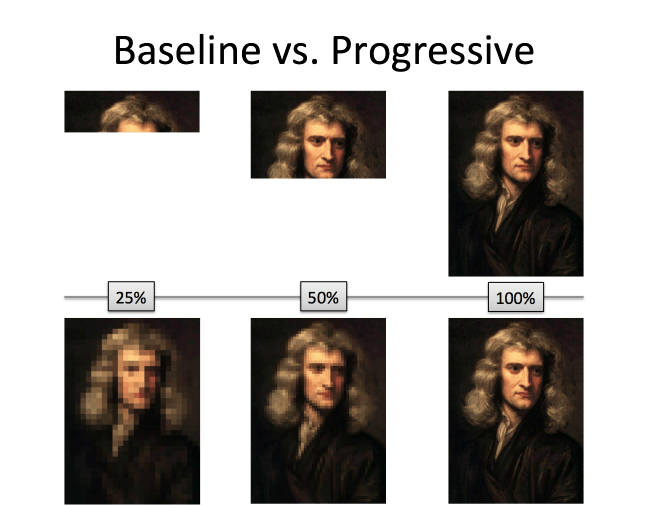Music Analogy In music: Appreciation (sounds good!), description (hum it), symbols (sheet music), performance (play it) In math: Appreciation (aha!), description (English), symbols (math), performance (calculate)
Appreciation vs. Performance We can enjoy listening to music even if we can't play it. We can think with calculus even if we can't (yet) compute with calculus.

## 2. Appreciating Calculus

Concept Key Analogy / Takeaway
Calculus in 1 minute See the world with X-Ray and Time-Lapse vision.Calculus in 10 minutes New viewpoints lead to insights: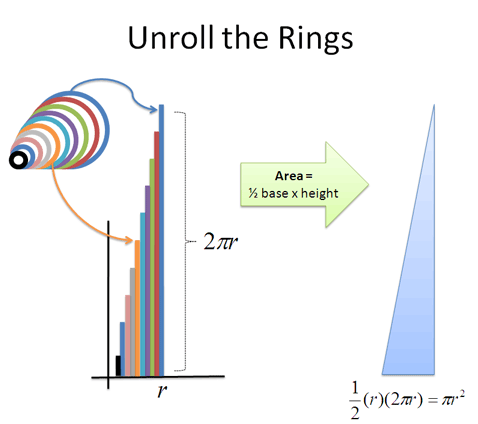Calculus explains X-Ray and Time-Lapse vision exist, they are opposites (splitting apart, gluing together) and any pattern can be analyzed.Arithmetic gives us add/subtract, multiply/divide, exponents/roots. "Calculus arithmetic" also has X-Ray (split apart) and Time-Lapse (glue together).
So what can I do with Calculus? See patterns at a deeper level and make predictions we couldn't before.
Example: Analyzing a Circle Build with rings, slices, or boards; each has tradeoffs.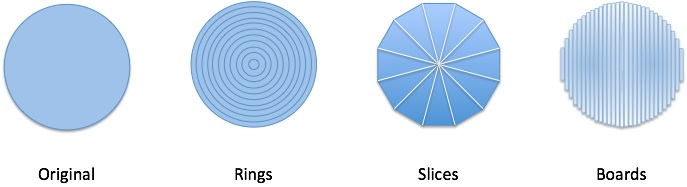Ring-by-ring View Organic growth, increasing effort.Slice-by-slice View Assembly-line, predictable progress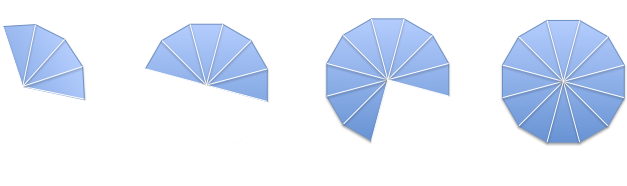Board-by-board View Robotic, never retracing3d versions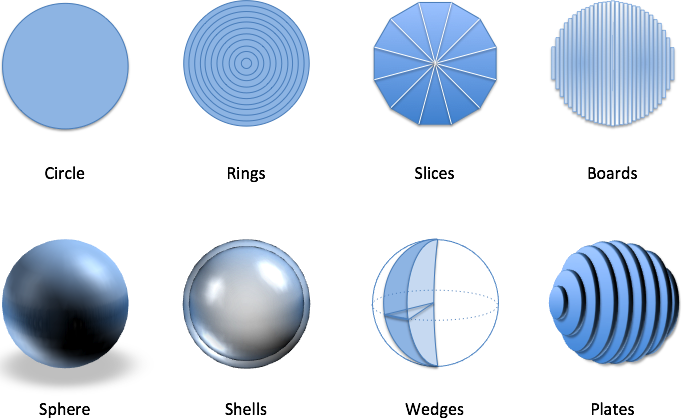How to think with calculus For your situation:
• X-Ray: What's the next thing that will happen? Any trend?
• Time-lapse: What outcome are these changes leading to?

## 3. Describing Calculus (English → Math)

Concept Key Analogy / Takeaway
Organize Our Descriptions Direction of slices in orange. Arrange slices side-by-side for easy comparison.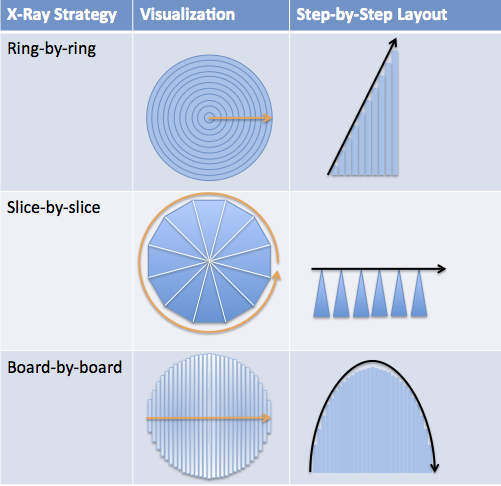Term: Derivative Creating a pattern of step-by-step slices along a path (i.e., rings, slices, boards, etc.).
Term: Integral Accumulating slices into a shape (what is being built up as we go?)
Notation Details Derivatives only require the direction we move when taking slices: frac(d)(dr) means slice along r's direction. Integrals require direction we glue together (dr), where we start/stop (0 to r), and the size of each slice (2 π r dr: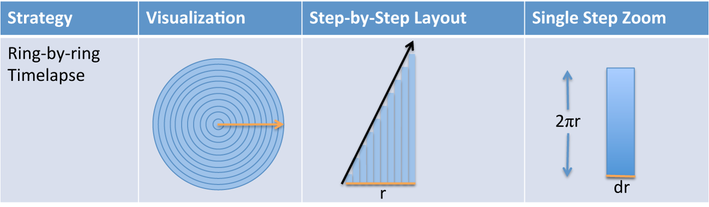Computer Calculation Wolfram Alpha can compute integrals/derivatives when asked: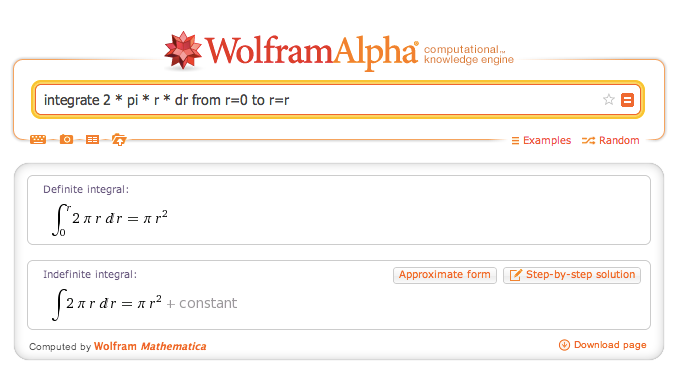Note about Abstraction Begin thinking about general patterns (x, x^2), not just specific shapes (a line, a square).
Lines Lines (f(x) = ax) change by a steady a each time, like building a fence.
Squares Squares (f(x) = x2) change by 2x + 1: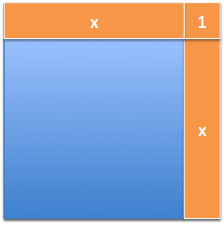## 4. Learning To Measure Change

Concept Key Analogy / Takeaway
Infinite processes can point to a result Analogy: fly going back and forth. Count its paths ("infinite"), or just the time traveled. Pixellated letters point to the smooth whole.
Analogy: Measuring Heart Rate Get on treadmill, hook up wires, run. The measurement is your heart rate under stress. Must then remove impact of wires.
The formal derivative Find change, then assume change had zero effect: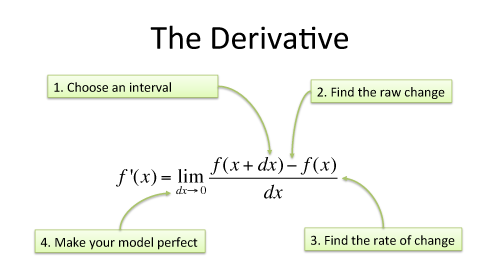Example 2x + dx overset(dx = 0) Longrightarrow 2x, so frac(d)(dx) x2 = 2x, with errors artifacts removed
Fundamental Theorem of Calculus The shortcut to computing the integral is finding a pattern that made the changes we're seeing.
Integrals are Reverse Engineered See patterns of steps and ask: what shape could have made this?L-shapes come from changing squares. Instead of adding (5 + 7 + 9 = 21), take the final square and subtract the starting one (25 - 4 = 21).

## 5. The Core Rules Of Calculus

Concept Key Analogy / Takeaway
Multiplication Rule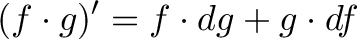$\displaystyle{(f \cdot g)' = f \cdot dg + g \cdot df}$ Grow a garden on two sides; ignore the corner.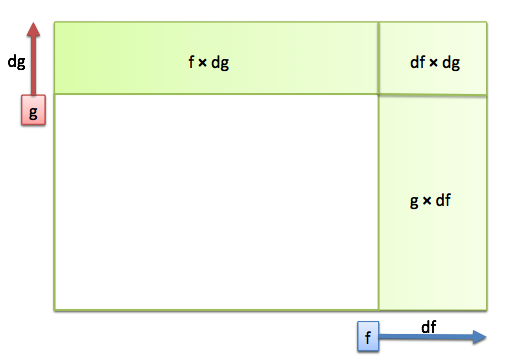Simple Division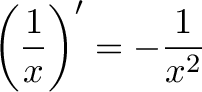$\displaystyle{ \left( \frac{1}{x} \right)' = -\frac{1}{x^2}}$ Splitting cake, new person enters (from halves to thirds).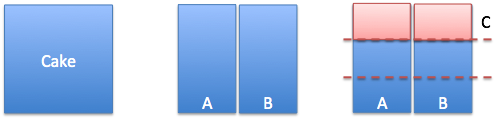Insight: Each perspective makes a contribution With 3 variables you have 3 perspectives to add: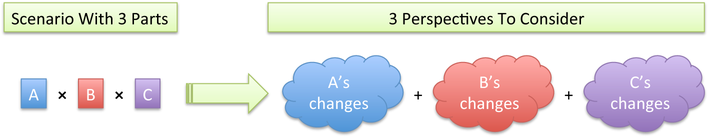Derivative of x · x · x has three identical perspectives (x2 + x2 + x2) or 3 x2.
Power Rule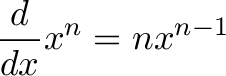$\displaystyle{\frac{d}{dx} x^n = n x^{n-1} }$ Each side has a point of view; I change, others are the same.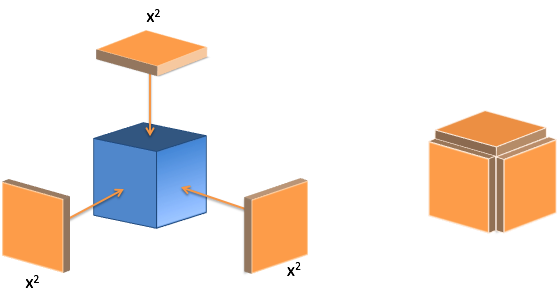Rule Summary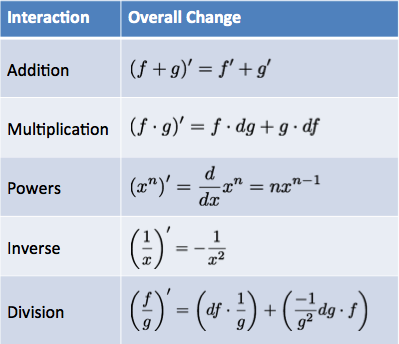## 6. Finding Archimedes' Formulas

Concept Key Analogy / Takeaway
Find the circle/sphere formulas X-Ray and Time-Lapse a single ring into the other shapes.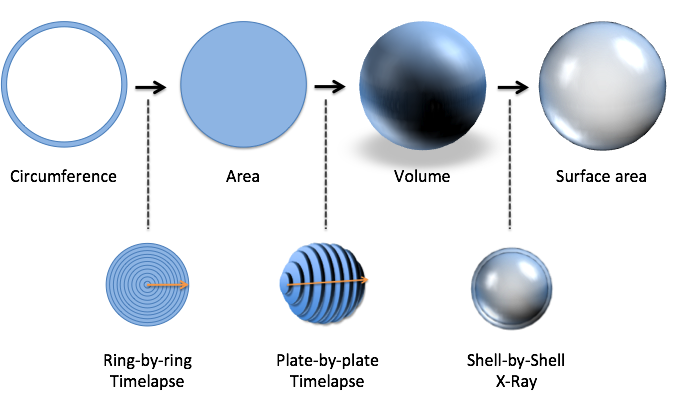Circumference to Area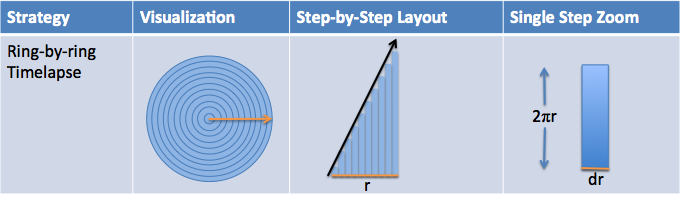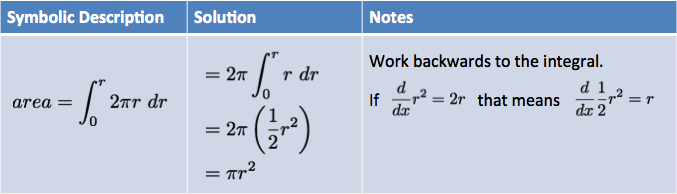Area to Volume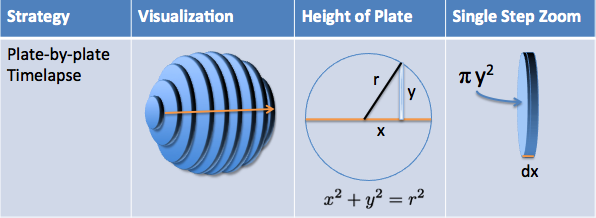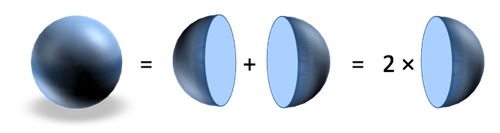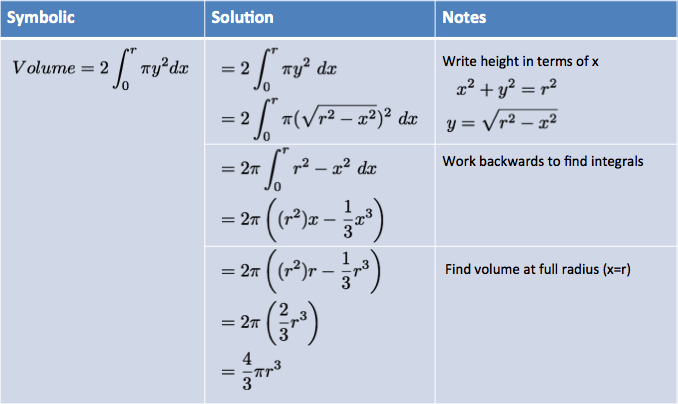Volume to Surface Area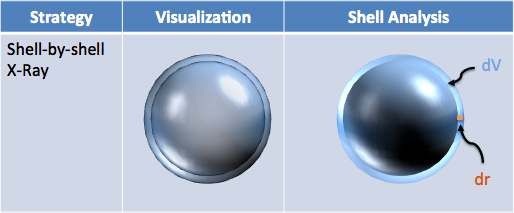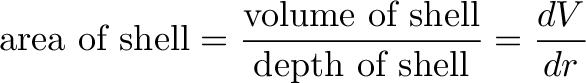$\displaystyle{\text{area of shell} = \frac{\text{volume of shell}}{\text{depth of shell}} = \frac{dV}{dr} }$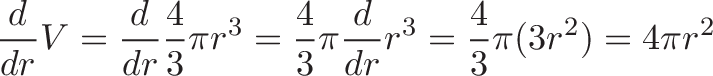$\displaystyle{\frac{d}{dr} V = \frac{d}{dr} \frac{4}{3} \pi r^3 = \frac{4}{3} \pi \frac{d}{dr} r^3 = \frac{4}{3} \pi (3 r^2) = 4 \pi r^2 }$
Historical Note Archimedes had a calculus mindset: he re-arranged discs, cylinders, cones, etc. to make "easy to measure" slices. The techniques you already know would make him tear up.